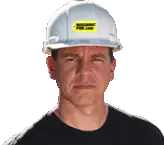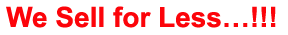# Construction Surveying - Earthwork

## Quiz Questions

 1. In road, railroad, and airfield construction, the movement of large volumes of earth (earthwork) is one of the most important construction operations. It requires a great amount of engineering effort. True False 2. The planning, scheduling, and supervising of earthwork operations are of minor importance in obtaining an efficiently operated construction project. True False 3. Earthwork computations do not involve the calculation of volumes or quantities, the determination of final grades, the balancing of cuts and fills, and the planning of the most economical haul of material. True False 4. __________________________are illustrated in figure 4-1. Typical cross sections for a railway track Typical cross sections for a roadbed 5. If the vertical cut or fill is small in comparison with the width, the surveyor may not use an exaggerated vertical scale to gain additional precision in plotting such sections. True False 6. The side slopes of a cross section are expressed by a ratio of horizontal distance to vertical distance. True False 7. The surveyor usually determines the slope by the design specifications based on the stability of the soil in cut or fill. True False 8. The stripper and the ___________________ methods are simple and give approximate results, while the other methods give results as accurate as the cross-section field data will permit. Geometric Counting squares Double-meridian-distance 9. To make a hasty approximation of a cross-section area plotted on cross-section paper, count the number of squares enclosed by the boundary lines of the section. Then divide the total number of counted squares by the number of square feet represented by a single square. True False 10. The _______________________ is also called a trapezoidal method. Double-meridian-distance method Counting squares method Geometric method Stripper method 11. Figure 4-4 illustrates ____________________. Cross section area by geometric method Cross section area by stripper method 12. To determine the area of a plotted cross section by strip measurements, subdivide the area into strips by vertical lines spaced at regular intervals. Measure the total length of these lines by cumulatively marking the length of each line along the edge of a stripper made of paper or plastic. Then, multiply the cumulative total of the average base lengths by the width of the strip. True False 13. DMD is an acronym for _______________. Double Meridian distance Double Meridian displacement 14. The stripper method gives a more precise value for a cross- section area than the double-meridian-distance (DMD) method. True False 15. The _________________ is based on the theory that the area of a right triangle equals one half of the product of the two sides. Geometric method Counting squares method Double-meridian-distance method Stripper method 16. What is the first step in the procedure to work out a DMD area? Select the far left station (D) as the first point Compute all the latitudes and departures. 17. The DMD of the last course is numerically equal to its departure but with the opposite sign (+14.0). True False 18. Figure 4-5 illustrates ____________________. Cross section area by geometric method Cross section area by DMD method 19. The surveyor does not use a polar planimeter to measure the area of a plotted figure. True False 20. The planimeters are of _________________ types. Two Three Four 21. The surveyor uses both types of the planimeters in the same way, with the exception that the fixed scale cannot be adjusted to yield a 1:1 ratio when tracing areas. True False 22. The planimeter (figure 4-6) touches the paper at how many points? Two Three Four 23. __________________ is usually classified as common excavation, loose rock, and solid rock. Excavated material Concrete 24. To measure plotted areas larger than the capacity of the planimeter, _____________the areas into sections and measure each separately. Multiply Divide 25. When there is an imbalance in the volume of cuts and fills in construction projects, it is often necessary to borrow the required fill dirt from borrow pits outside the construction limits but near the fill site. True False